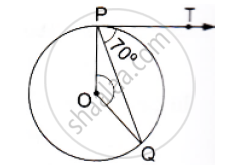# If Pt is a Tangent to a Circle with Center O and Pq is a Chord of the Circle Such that ∠Qpt = 70°, Then Find the Measure of ∠Poq. - Mathematics

If PT is a tangent to a circle with center O and PQ is a chord of the circle such that ∠QPT = 70°, then find the measure of ∠POQ.#### Solution

We know that the radius and tangent are perpendicular at their point of contact.
∴ ∠OPT = 90°
Now, ∠OPQ = ∠OPT - ∠TPQ = 90° -70°  = 20°
Since, OP = OQ as both are radius
∴ ∠OPQ = ∠OQP = 20° (Angles opposite to equal sides are equal)
Now, In isosceles Δ POQ
∠POQ + ∠OPQ + ∠OQP = 180°       (Angle sum property of a triangle)

⇒ ∠POQ =180° - 20°  = 140°

Concept: Concept of Circle - Centre, Radius, Diameter, Arc, Sector, Chord, Segment, Semicircle, Circumference, Interior and Exterior, Concentric Circles
Is there an error in this question or solution?